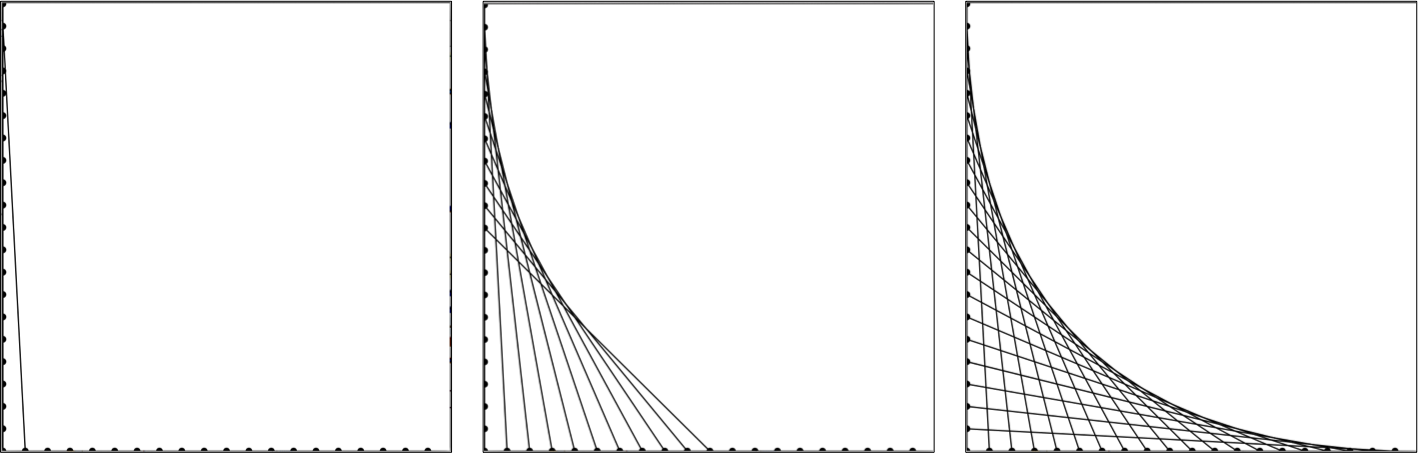By repeatedly drawing lines, we can make a curved looking shape. Consider the example below where we imagine dots evenly space along the bottom and the left of a canvas. If we connect the topmost dot on the left edge to the left most dot on the right edge, we would be a line like the picture of the far left. If we then connect the next 10 dots we get a picture in the middle and if we connect all dots we get the picture on the right.Write a program that creates the "curve" in the above right image using straight lines.

## Solution

```public class StringArt extends GraphicsProgram {

/** The size of the screen **/
public static final int APPLICATION_WIDTH = 400;
public static final int APPLICATION_HEIGHT = 400;

/** The number of pixels between end points of the line **/
public static final int LINE_SPACING = 20;

/** The number of lines we will have to draw **/
public static final int NUM_LINES = APPLICATION_WIDTH / LINE_SPACING;

public void run() {
for(int i = 0; i < NUM_LINES; i++) {
int offset = i * LINE_SPACING;
int startX = offset;
int startY = getHeight();
int endX = 0;
int endY = offset;
GLine line = new GLine(startX, startY, endX, endY);